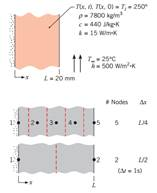### Create an Account

Already have account?

### Forgot Your Password ?

Home / Questions / In Section 55 the oneterm approximation to the series solution for the temperature distrib...

# In Section 55 the oneterm approximation to the series solution for the temperature distribution was developed for a plane wall of thickness 2L that is initially at a uniform temperature and

In Section 5.5, the one-term approximation to the series solution for the temperature distribution was developed for a plane wall of thickness 2L that is initially at a uniform temperature and suddenly subjected to convection heat transfer. If Bi(a) Determine the midplane, T(0, t), and surface, T(L, t), temperatures at t = 100, 200, and 500 s using the one-term approximation to the series solution, Equation 5.43, What is the Biot number for the system?

(b) Treating the wall as a lumped capacitance, calculate the temperatures at t = 50, 100, 200, and 500 s. Did you expect these results to compare favorably with those from part (a)? Why are the temperatures considerably higher?

(c) Consider the 2- and 5-node networks shown schematically. Write the implicit form of the finitedifference equations for each network, and determine the temperature distributions for t = 50, 100, 200, and 500 s using a time increment of ∆t = 1 s. You may use IHT to solve the finite-difference equations by representing the rate of change of the nodal temperatures by the intrinsic function, Der(T, t). Prepare a table summarizing the results of parts (a), (b), and (c). Comment on the relative differences of the predicted temperatures.

Jun 27 2020 View more View Less

#### Answer (Solved)Subscribe To Get Solution